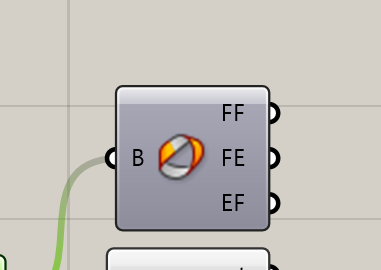# Mesh Topology, Face Edge Neighbours C# or Python

Hello Guys

please tell me, is it possible somehow using a python or from a sharpe to list the numbers of neighboring lines with a network edge in the tree lists, and similarly, by analogy with the Brep Topology node?a similar question is considered for bodies in this topic

could find using for example a plug-in mesh + it is possible to see neighboring faces

MeshFaceEdgeNeighbours.gh (20.5 KB)

I might be misunderstanding the problem, but if you’re trying to compute node valences for a network of connecting lines, you can get their end-points and count their occurrence. Like so:

2 Likes

Check out the Topologizer plugin by Daniel Piker:

1 Like

I probably didn’t exactly put it, is it possible to find neighboring edges on the mesh
is it possible to make an analog node only for the mesh

analog only for the mesh

2 Likes

Not sure I understand either, but if you just want to recreate the brep topology for a mesh:

``````DataTree<int> edgeFaceAdjacency = new DataTree<int>();
for (int e = 0; e < mesh.TopologyEdges.Count; e++)
{
mesh.TopologyEdges.GetConnectedFaces(e),
new GH_Path(e));
}

for (int f = 0; f < mesh.Faces.Count; f++)
{
new GH_Path(f));
}

for (int e = 0; e < mesh.TopologyEdges.Count; e++)
{
var connectedFaces = mesh.TopologyEdges.GetConnectedFaces(e);
foreach (int f in connectedFaces)
{
}
}
``````

Or just use the API methods mentioned aboveProbably the last part is a bit a workaround and the API method keeps the sorting consistent.

2 Likes

Given the opportunity and using Methods exposed in the R SDK this is how to do (C#) the 7 (out of the 9) mesh connectivity trees (3 classes of collections [V,E,F] meaning 9 possible combos). For the rest 2 you’ll need a couple of lines more.

MTV, MTE, MF are MeshTopologyVertices, MeshTopologyEdges and MeshFaces public collections (as they are the conn Trees).

1 Like

I have a question. How do you get all the curves at each node？

This is called VE Connectivity.

First get the EV and VV [using TopologyVertices indexing - NOT Vertices indexing] Connectivity (R Common has Methods for these).

Then do this:

As a challenge try to get the 9 Connectivity Trees (3 classes: TopoVertices[V], TopoEdges[E] and Faces[F] … thus 9 possible combos: VV, VE, VF, EV, EE, EF, FV, FE, FF).